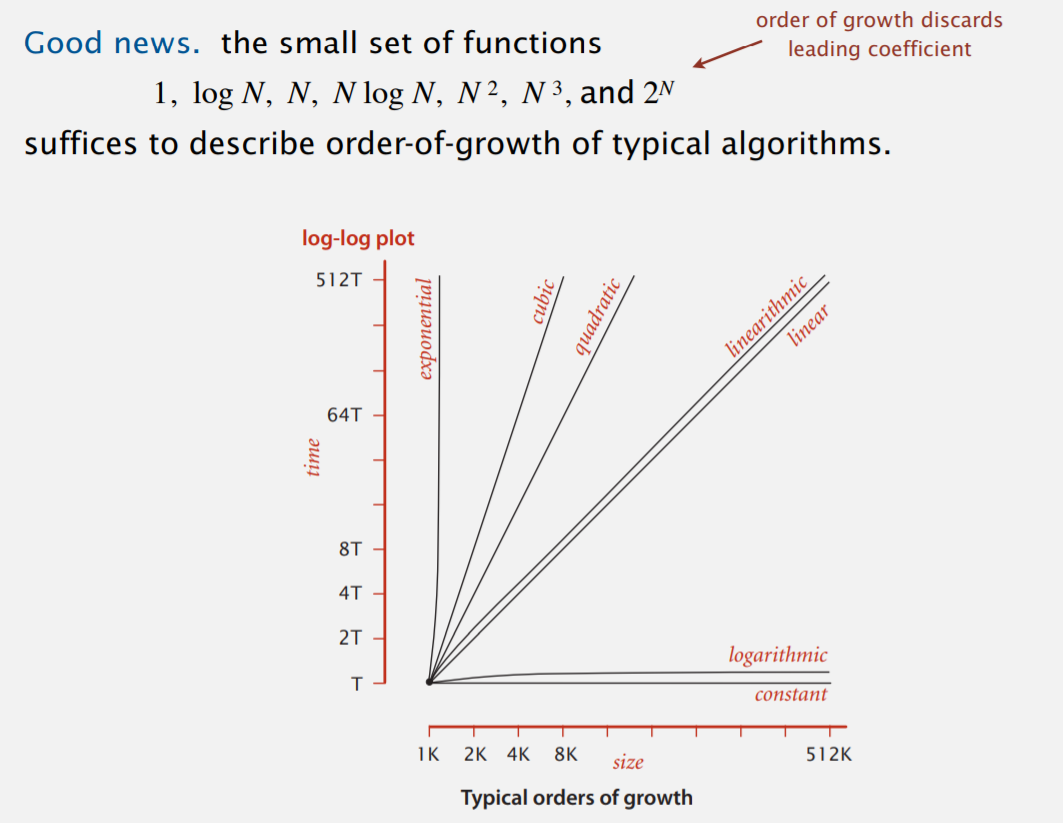# 算法时间复杂度

## 2. 分类• exponential：指数复杂度
• cubic$N^3$
• quadratic$N^2$
• linearithmic$N\log N$
• linear$N$
• logarithmic$\log N$
• constant$C$

## 3. 符号

$N\log N$ 为例：

• $\Theta(N \log N)$：表示时间复杂度渐近为 $N \log N$

• $O(N \log N)$：表示时间复杂度小于等于 $N \log N$

• $\Omega(N \log N)$：表示时间复杂度大于等于 $N \log N$